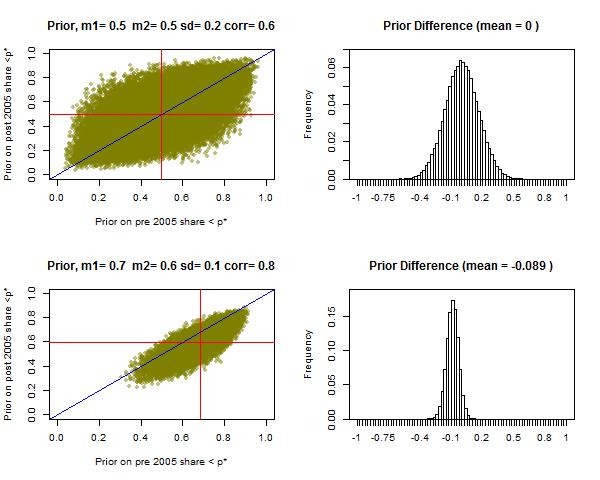# New Year Medical Stats Puzzle

Task: Guess the effects of registration on medical ps. [See short description here http://www.columbia.edu/~mh2245/p/2pager.pdf]

We want to figure out whether the introduction of registration requirements affected the types of statistics published in medical journals. We have the data but we have not looked at it yet. To avoid doing the kind of data fishing that many hope registration will prevent we are registering our analysis plan before we start. See details here http://www.columbia.edu/~mh2245/p/

One innovation of this project is that we want to figure out what we learn about registration relative to current beliefs. What do you expect we will find? Has there been an effect or not?

Here you can give us your best guess for:
(i) the difference in the share of p values just below 0.05 before and after 2005 and
(ii) the difference in the share of all p values below 0.001 before and after 2005.

And for the more intrepid we ask that you also provide a full specification of your *prior* (current) beliefs as well as an indication of your belief about the appropriate classical test.
This is a required question
This is a required question
This is a required question

## The two prize questions: Guess d1 and d2

This is a required question
This is a required question

## What are the implications for registration if we find nothing?

We are interested in the causal effect of the 2005 registration requirements set by medical journals, but all we can measure is the change in the distribution of published p values. This is useful information if we think that these are likely to shift if there was a causal effect and not likely to shift if there was not. But it is possible that the distribution of ps does not shift even if there is an effect (since registration could affect the types of studies undertaken and not just how they are analyzed and published) and it is possible that it does shift even if there is no effect (especially for our second test since other things might also have changed between the pre and post periods). So this leaves interpretation ambiguity. Tell us now how you will interpret our findings:
This is a required question
This is a required question
This is a required question
This is a required question
This is a required question

## Four questions about you

This is a required question
This is a required question
This is a required question
This is a required question

## Free text

Do you have any other comments eg regarding strategies to analyze the data or interpret the findings?
This is a required question

## Last Questions About Your Priors (Hard to answer but very useful!)

We now ask you to report your priors about the underlying probabilities of producing published results above and below the key thresholds in each period. The interest here is not in the actual share in the different categories (d_1 and d_2) but in the but the underlying data generating process. This is a difficult business and we know that this is very difficult. To make it as simple as possible though we have set things up so that you can report in terms of four parameters, "mu0" (average probabilities before 2005), "mu1" (after after 2005), "sd" (uncertainty), and "corr" (correlation). Together these four parameters can describe a joint probability distribution over pi0 and pi1 where pi0 is the probability that a given p value will be below a threshold pre 2005 and pi1 the probability that it will be below post 2005. We provide a tool to help see how these parameters map onto a prior distribution here: https://macartan.shinyapps.io/delta_priors/ and some illustrations below. The four numbers correspond closely (but not exactly) to the mean of the prior of the share below the critical value pre 2005; the mean below post 2005, the (common) standard deviation of these two, and the correlation between them. eg if you were nearly certain that the share would be .5 in each period and that it would be the same in both periods you might have m0=m1=.5, sd=.01, corr=1. The illustrations below give some examples of possible prior distributions and the implied prior distribution on d.

## Illustration of Priors## Priors for reporting around the 0.05 threshold

Please provide 4 numbers (mu1, mu2, sd, corr) to describe your priors over the share of reported ps below 0.05 pre 2005 and the share below post 2005. Here we condition on ps in the neighborhood of 0.05 only (specifically those with implied z-stats between 1.66 and 2.26). For help on how these numbers map to priors see https://macartan.shinyapps.io/delta_priors/
Numbers between 0 and 1 please
This is a required question
Must be a number less than or equal to 1
This is a required question
Numbers between 0 and 1 please
This is a required question
Between -1 and 1 please
This is a required question

## Priors for reporting around the 0.001 threshold

Same as above: please provide 4 numbers (mu0, mu1, sd, corr) to describe your priors over the share of reported ps below 0.001 pre 2005 and the share below post 2005. For help on how these numbers map to priors see https://macartan.shinyapps.io/delta_priors/
Numbers between 0 and 1 please
This is a required question
Must be a number less than or equal to 1
This is a required question
Must be a number less than or equal to 1
This is a required question
Between -1 and 1 please
This is a required question